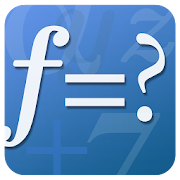FX Math Problem SolverEveryone
972
Offers in-app purchases
FX Math Solver is a comprehensive math software, based on an automatic mathematical problem solving engine,
and ideal for students preparing term math exams, ACT, SAT, and GRE:

- Over 1,500 sample math problems and fully animated solution steps
- Scientific calculator supported
- Graphing calculator supported
- Automatic problem solving and generation of fully animated step-by-step procedures for problems typed in by users
- User friendly math problem expression editor (WYSIWYG mode)

Math Solver covers problems at the level of Pre-Algebra, Algebra1, Algebra2, and Calculus courses:

- Number operations: add, subtract, multiply, divide
- Prime factorization
- Mixed number
- Complex number

- Basic expression simplification:
- polynomial - factorization, long-division
- rational
- exponential and log/ln
- sin, cos, tan, ..
- Matrix
- Equations, system of equations
- Inequalities
- Function
- Graphing
- Limit
- Differentiation
- Integral
Collapse

Reviews

Review Policy
4.1
972 total
5
4
3
2
1

Updated
May 11, 2019
Size
38M
Installs
100,000+
Current Version
2.1
Requires Android
4.4 and up
Content Rating
Everyone
In-app Products
\$4.90 per item
Permissions
Offered By
Euclidus Inc
Developer
5042 Wilshire Blvd #360 Los Angeles, CA 90036 USA